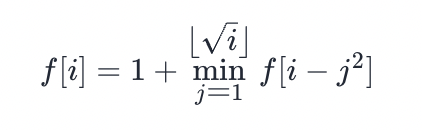# Leetcode 题目解析：279. 完全平方数# 三 题目解析

## 3.1 示例

输入：n = 12输出：3 解释：12 = 4 + 4 + 4

## 3.2 动态规划解法Java 版代码如下：

public int numSquares(int n) {        int[] f = new int[n + 1];        for (int i = 1; i <= n; i++) {            int minn = Integer.MAX_VALUE;            for (int j = 1; j * j <= i; j++) {                minn = Math.min(minn, f[i - j * j]);            }            f[i] = minn + 1;        }        return f[n];    }## 评论